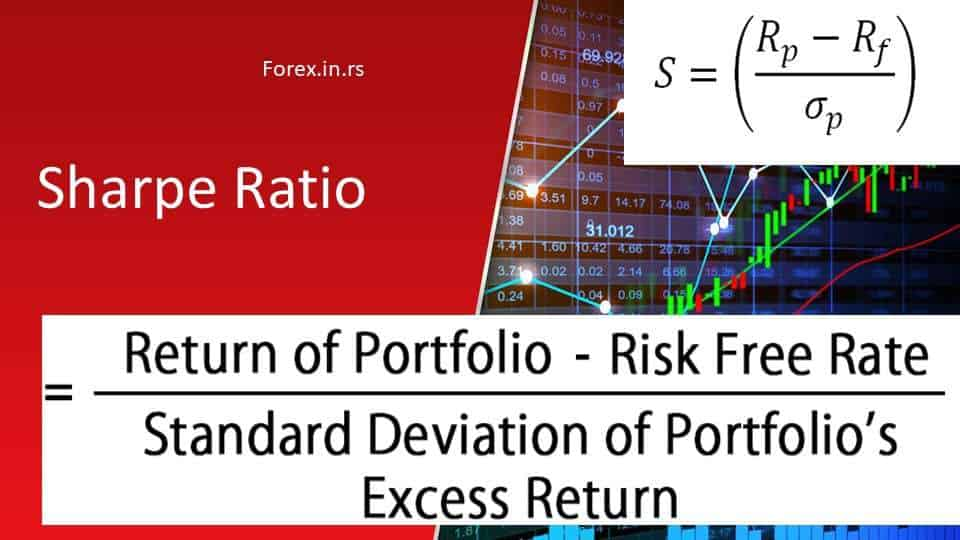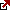# What is the Sharpe ratio?

In 1996, the Sharpe ratio was created by William Sharpe. Ever since then, it’s been used as the referenced risk in finance. It is that popular because of its ease of use. In 1990, Professor Sharpe was awarded a Nobel prize in Economic Science for his effort towards CAPM. And this later contributes to its credibility.

## What is the Sharpe ratio?

The Sharpe ratio (Sharpe index or the Sharpe measure or reward-to-variability ratio) explains an investment’s performance. It is represented as the difference between the average returns of the investment and the risk-free return, divided with volatility. The volatility or standard deviation of the investment is measured by an asset or portfolio’s price fluctuations.

What is a good Sharpe ratio for a fund?
Usually, the good Sharpe ratio is above 1 for most investors. A Sharpe ratio higher than 2 is rated as very good, and the Sharpe ratio above 3.0 is considered excellent.

So, how to calculate a Sharp ratio?The Sharpe ratio formula is given here as:

S(x) = (Rx – Rf)/ StdDev(X)

Where;
X= the investment, Rx = x average return rate
Rf = best available rate of return, StdDev(X) = standard deviation
A. Rx, i.e., return if normally shared, maybe per year, month week, or per day. This then reveals the shortcoming of the ratio; all returns on assets are not often normally shared.

As we can see, the Sharpe ratio is an excellent measure of an investment’s performance. Sharpe ratio and Payoff ratio are excellent tools to analyze and compare portfolios.

How to calculate Sharp ratio video:

How to calculate Sharp ratio using Excel:

Kurtosis: This is the peakedness or flatness of a frequency distribution
skewness graph: This is a headache to the ratio because standard deviation may not be an option when these problems occur. Sometimes, it can be risky to apply this formula when returns are not in the normal distribution.
B. The risk-free rate of return (rf): This is applied to reveal if one is appropriately rewarded for taking a further risk. Normally, the most archaic in U.S T-bill is the risk-free rate on return. Though this kind of security is more stable, risk-free security has been debated by some that are supposed to match the period of comparable investment.
For instance, equities remain an asset that persists for a long period. Perhaps we should not compare them with the long period risk-free asset present: the government issued IPS? Applying IPS that can persist for a long time would result in different ratio values because normally, under interest rate clime, T-bills have lesser returns than IPS.

For example, a decade index returned to three percent of the year 2017 on inflation-protected securities was reported by BARCLAYS US TREASURY.
In contrast, within the same duration of time, a 7.4% index returned was reported by S and P 500. One may debate that investors giving preference to equities over bonds were fairly rewarded. If the Sharpe ratio has a bond index of 1.16% against a 0.38% equity index, equities assets are riskier.

STANDARD DEVIATION
Since we’ve gotten excess return by subtracting the risk-free rate of return from the risky asset return, there’s a need to divide the answer by the standard deviation of the calculated risky asset. As stated above, as the number rises, the investment gets better.
The way of sharing the returns is Sharpe’s ratio of soft rot. Bell curves were employed for convenience mathematically according to Nassim Nicholas and Mandelbrot in 2005.
Bell curves also never take a big shift into account.
Nevertheless, leverage does affect the ratio of the standard deviation is small.
The return and the standard deviation can be two times their original size without hitches.
There’s an issue if the denominator is becoming too high. For instance, a stock price that’s supported 10 to 1 without much ado may drop by 10%; this corresponds to a 100% fall in startup capital.

## The Sharpe ratio and the risk

Knowing the relationship between risk and Sharpe ratio is from calculating the denominator, which is also referred to as total risk. Variance is calculated by squaring the standard deviation, i.e., the denominator.
Why did Sharpe pick a denominator to balance excesses? Since we are aware that Markowitz comprehends variance- which is one the way of measuring dispersion in statistics, something the investor detests. The standard deviation unit is the same as the measured data series.
Here are the examples showing reasons why investors should be mindful of variance:
Any investors are at liberty to pick 3 portfolios, expecting returns of 10% on all in ten years.
The table given below depicts the given expectation. Investment horizon returns gained shown per annum.
From the table, it’s shown that standard deviation shifts return from expectation.
In the absence of risk standard deviation is zero. This means the expectation is equal to returns.

Expected Average Returns
Year Portfolio A Portfolio B Portfolio C
Year 1 10.00% 9.00% 2.00%
Year 2 10.00% 15.00% -2.00%
Year 3 10.00% 23.00% 18.00%
Year 4 10.00% 10.00% 12.00%
Year 5 10.00% 11.00% 15.00%
Year 6 10.00% 8.00% 2.00%
Year 7 10.00% 7.00% 7.00%
Year 8 10.00% 6.00% 21.00%
Year 9 10.00% 6.00% 8.00%
Year 10 10.00% 5.00% 17.00%
Average Returns 10.00% 10.00% 10.00%
Annualized Returns 10.00% 9.88% 9.75%
Standard Deviation 0.00% 5.44% 7.80%

## How to use the Sharpe ratio?

In this video below, we will see the portfolio Sharpe ratio explanation:

Sharpe ratio may be used to measure return and adjust risk to compare the investment manager’s performance.
For instance, say one investment manager makes fifteen percent returns and the other twelve percent return. The latter looks excellent in performance. Though the latter took higher risks than the former, the former risk-adjusted return may be better. To buttress this example, a 5% free-risk rate is available; the first managers have eight percent standard deviation, five percent for the second manager. The first would realize the Sharpe ratio of 1.25. then 1.4 goes to the second manager; this is better compared to the first manager. Hence as a result of this calculation, the second manager depending on the risk-adjusted, got better returns.
NOTE:
Ratio 1: Good
Ratio2: Very good
Ratio3: Excellent

What does negative Sharpe ratio mean

A negative Sharpe ratio means that a manager or portfolio’s performance is below the risk-free rate and can endure for long periods of time. Interestingly, the negative Sharpe ratio is problematic to evaluate during the volatile market. A portfolio with a small negative excess return can be punished if the volatility associated with it is large.

So, is a negative Sharpe ratio bad? A negative Sharpe ratio is a bad characteristic for a portfolio because it shows that it has underperformed its benchmark.

What is the Sharpe ratio of the S&P 500?
In the 21. In the last 20 years, the average annual Sharpe ratio for the S&P 500 has been 1.2, with frequent periods of much higher and lower levels.

## Bottom line

Evaluation of risk and compensation is done together while making investment decisions, and this is the main focus of Modern Portfolio Theory.
Generally, variance or its square root drifts compensation away from the investor. So to choose an investment, it is advisable to deal with risk and reward together. Sharpe ratio will help make investment decisions that will yield bigger returns even when the risk is considered.

Latest posts by Fxigor (see all)

Fxigor

Igor has been a trader since 2007. Currently, Igor works for several prop trading companies. He is an expert in financial niche, long-term trading, and weekly technical levels. The primary field of Igor's research is the application of machine learning in algorithmic trading. Education: Computer Engineering and Ph.D. in machine learning. Igor regularly publishes trading-related videos on the Fxigor Youtube channel. To contact Igor write on: igor@forex.in.rs

Trade gold and silver. Visit the broker's page and start trading high liquidity spot metals - the most traded instruments in the world.